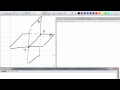Course

4.95

(21)

Certificate

This course is free today and always will be, from start to finish you will pay nothing.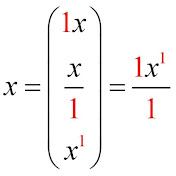Teacher

Algebra: Structure and Method, Book 1 Geometry by Jurgensen

Course content preview

0h32m

0h34m

0h23m

0h06m

0h35m

0h24m

0h35m

0h29m

0h29m

0h26m

0h31m

0h21m

0h33m

0h23m

0h31m

0h23m

0h11m

0h30m

0h08m

Geometry 4.1 Confruent Figures

0h14mWatch the 1st video of the course

Do not worry, it is free!

Course content0h32m

Geometry 1.2

Geometry 1.2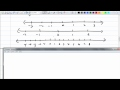0h34m

Geometry 1.3

Geometry 1.3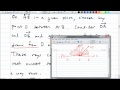0h23m

geometry 1.4 angles

geometry 1.4 angles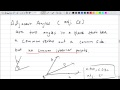0h06m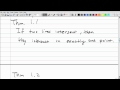0h35m

geometry 1.5 postulates and theorems

geometry 1.5 postulates and theorems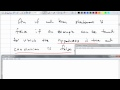0h24m

geometry 2.1 if and then statements

geometry 2.1 if and then statements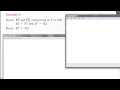0h35m

geometry 2.2 Properties from Algebra

geometry 2.2 Properties from Algebra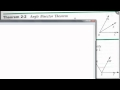0h29m

geometry 2.3 proving theorems

geometry 2.3 proving theorems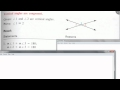0h29m

Geometry 2.4 special pairs of angles

Geometry 2.4 special pairs of angles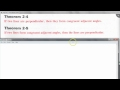0h26m

Geometry 2.5 perpendicular lines

Geometry 2.5 perpendicular lines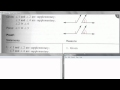0h31m

Geometry 2.6 planning a proof

Geometry 2.6 planning a proof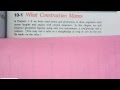0h21m

geometry constructions 1~6

geometry constructions 1~6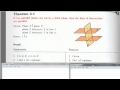0h33m

Geomtry 3.1 Definitions

Geomtry 3.1 Definitions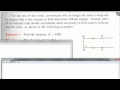0h23m

Geometry 3.2 properties of parallel lines

Geometry 3.2 properties of parallel lines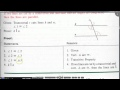0h31m

Geometry 3.3 proving lines parallel

Geometry 3.3 proving lines parallel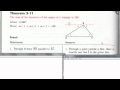0h23m

Geometry 3.4 angles of a triangle (day1)

Geometry 3.4 angles of a triangle (day1)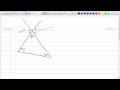0h11m

Geometry 3.4 angles of a triangle (day2)

Geometry 3.4 angles of a triangle (day2)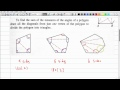0h30m

Geometry 3.5 Angles of a ploygon

Geometry 3.5 Angles of a ploygon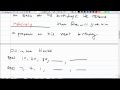0h08m

Geometry 3.6 Inductive Reasoning

Geometry 3.6 Inductive Reasoning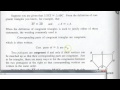0h14m

Geometry 4.1 Confruent Figures

Geometry 4.1 Confruent Figures